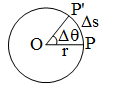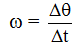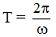# Physics

### Chapter : 1. Motion

#### Circular Motion

When a body moves in such a way that its distance from a fixed point always remains constant, then its motion is said to be the circular motion.
Uniform Circular Motion :
If the radius vector sweeps out equal angles in equal times, then its motion is said to be uniform circular motion.In uniform circular motion speed remains const.
Linear velocity, being a vector quantity, its direction changes continuously.
The direction of velocity is along the tangent at every point.
Angular Velocity :A vector quantity
Direction is perpendicular to plane of rotation
Note : If the particle is revolving in the clockwise direction then the direction of angular velocity is perpendicular to the plane downwards. Whereas in case of anticlockwise direction, the direction will be upwards.
Unit of Angular Velocity is Radian/sec.
In uniform circular motion the direction of angular velocity is along the axis of rotation which is constant throughout.
Angular velocity remains constant in magnitude as well as in direction.
v = rω where r = radius of the circle.
Centripetal Acceleration
In uniform circular motion the particle experiences an acceleration called the centripetal acceleration.The direction of centripetal acceleration is along the radius towards the centre.
Centripetal force :
Always acts towards centre.
Centripetal force is required to move a particle in a circle.
Because Fc is always perpendicular to velocity or displacement, hence the work done by this force will always be zero.
Note :
Circular motion in horizontal plane is usually uniform circular motion.
Remember that equations of motion are not applicable for circular motion.
Time period :
It is the time taken to complete one complete revolution.
In one revolution, angle subtended is 2π and if T is time period, then the angular velocity is given byorFrequency :
Frequency is defined as the number of revolutions per second.
i.e.